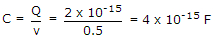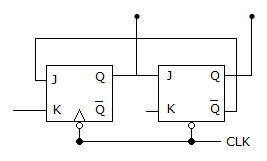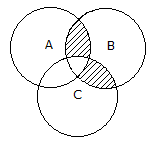# Online Electronics and Communication Engineering Test - Digital Electronics Test 1Loading Test...

Instruction:

• This is a FREE online test. DO NOT pay money to anyone to attend this test.
• Total number of questions : 20.
• Time alloted : 30 minutes.
• Each question carry 1 mark, no negative marks.
• DO NOT refresh the page.
• All the best :-).

1.

A.
 binary states 1000 to 1111B.
 binary states 0000 to 0011C.
 binary states 1010 to 1111D.
 binary states 1111 to higherExplanation:

A decade counter counts from 0 to 9. It has 4 flip-flops. The states skipped are 10 to 15 or 1010 to 1111.

2.

A ring counter with 5 flip flops will have

A.
 5 statesB.
 10 statesC.
 32 statesD.
 infinite states3.

The minimum number of NAND gates required to implement the Boolean function A +AB + ABC is equal to

A.
 0B.
 1C.
 4D.
 7Explanation:

A + AB +A B C = A + AB ( 1 + C) = A + AB = A(1 + B) = A.

4.

The number of counter states which an 8 bit stair step A/D converter has to pass through before conversion takes place is equal to

A.
 1B.
 8C.
 255D.
 256Explanation:

28 = 256.

5.

A dynamic RAM cell which holds 5 V has to be refreshed every 20 ms so that the stored voltage does not fall by more than 0.5 V. If the cell has a constant discharge current of 0.1 pA, the storage capacitance of cell is

A.
 4 x 10-6 FB.
 4 x 10-9 FC.
 4 x 10-12 FD.
 4 x 10-15 FExplanation:

Q = 20 x 10-3 x 0.1 x 10-12 = 2 x 10-15 C and.

6.

The modulus of counter in the given figure isA.
 1B.
 2C.
 3D.
 4Explanation:

Third clock pulse resets the counter to 00 state. Hence mod is 3.

7.

Which of the following is not a characteristic of a flip flop?

A.
 It is a bistable deviceB.
 It has two outputsC.
 It has two outputs are complement of each otherD.
 It has one input terminalExplanation:

Flip-flop has more than 1 input.

8.

Y = A + A B is the same as

A.
 Y = ABB.
 Y = A + BC.
 Y = A + BD.
 Y = A + BExplanation:

Verify by preparing truth table.

9.

A microprocessor with a 16 bit address bus is used in a linear memory selection configuration. Address bus lines are directly uses as chip selects of memory chips with 4 memory chips the maximum addressable memory space is

A.
 64 kB.
 16 kC.
 8 kD.
 4 k10.

Assertion (A): A high density IC has more diodes, transistors and resistors per unit surface area.

Reason (R): A high density IC can handle high voltages.

A.
 Both A and R are correct and R is correct explanation of AB.
 Both A and R are correct but R is not correct explanation of AC.
 A is true, R is falseD.
 A is false, R is true11.

The boolean expression for shaded area in the given figure isA.
 AB + ACB.
 ABC + ABCC.
 ABC + ABCD.
 None of these12.

Which of the following is not a specification of D/A and A/D converters?

A.
 GainB.
 DriftC.
 SpeedD.
 Accuracy13.

Which of the following is susceptible to race condition?

A.
 R-S latchB.
 D latchC.
 Both R - S and D latchesD.
 None of the above14.

For a NAND SR latch of input is the normal resting state of inputs is

A.
 S = R = 1B.
 S = 0, R = 1C.
 S = 1, R = 0D.
 S = R = 015.

Reason (R): The carry look ahead adder generates the carry and sum digits directly.

A.
 Both A and R are correct and R is correct explanation of AB.
 Both A and R are correct but R is not correct explanation of AC.
 A is true, R is falseD.
 A is false, R is true16.

The high voltage level of a digital signal in positive logic is

A.
 1B.
 0C.
 either 1 or 0D.
 -117.

In a sequential circuit the output at any instant depends on

A.
 present inputs onlyB.
 past inputs onlyC.
 past outputs onlyD.
 past output and present input18.

Which of the- following display consumes least amount of power?

A.
 LCDB.
 LEDC.
 Fluorescent displayD.
 All display consume same power19.

If one wants to design a binary counter, preferred type of flip-flop is

A.
 D-typeB.
 SR typeC.
 LatchD.
 JK type20.

Assertion (A): A presettable counter can be preset to any desired starting point

Reason (R): The maximum frequency of a ripple counter depends on the modulus.

A.
 Both A and R are correct and R is correct explanation of AB.
 Both A and R are correct but R is not correct explanation of AC.
 A is true, R is falseD.
 A is false, R is true